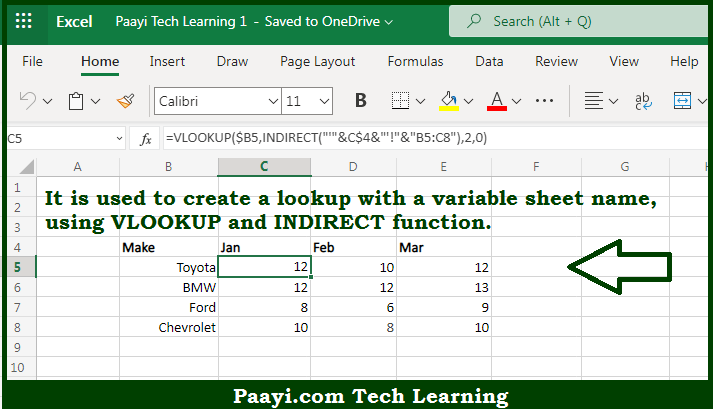# Learn How to Lookup With Variable Sheet Name in Microsoft Excel

Written by | 0 Comments | 272 Views

In this article, you will learn how to evaluate things based on the LOOKUP function in Microsoft Excel using a single/combination(s) of functions. You will also know how to Lookup With Variable Sheet Name and see the generic formula.

Learn How to Lookup With Variable Sheet Name in Microsoft Excel

The main purpose of this formula is to create a Lookup with a variable sheet name. Here we will learn how to perform a lookup for the variable sheet name in the given data range in the workbook in Microsoft Excel. That implies, with the help of a formula based on the VLOOKUP and INDIRECT functions you can able to create a lookup with a variable sheet name. So, with the help of this formula, you can able to perform a lookup for the variable sheet name in the given data range in the workbook in Microsoft Excel.

General Formula to Lookup With Variable Sheet Name

=VLOOKUP(val,INDIRECT("'"&sheet&"'!"&"range"),col,0)

The Explanation to Lookup With Variable Sheet NameSo we know that with the help of the given formula above you can able to create a Lookup with a variable sheet name. Here we will learn how to perform a lookup for the variable sheet name in the given data range in the workbook in Microsoft Excel. As we know the prime goal here is to create a lookup formula with a variable sheet name. That implies a formula that uses the sheet name in a way that can be changed by referring to up a value on the worksheet.

The INDIRECT function is the key to the solution, which evaluates text as a worksheet reference. This makes it possible to build a formula to assemble a reference as text using concatenation and use the resulting text as a valid reference. So now you have learned how to perform the lookup for the variable sheet name in the given data range in the workbook in Microsoft Excel.